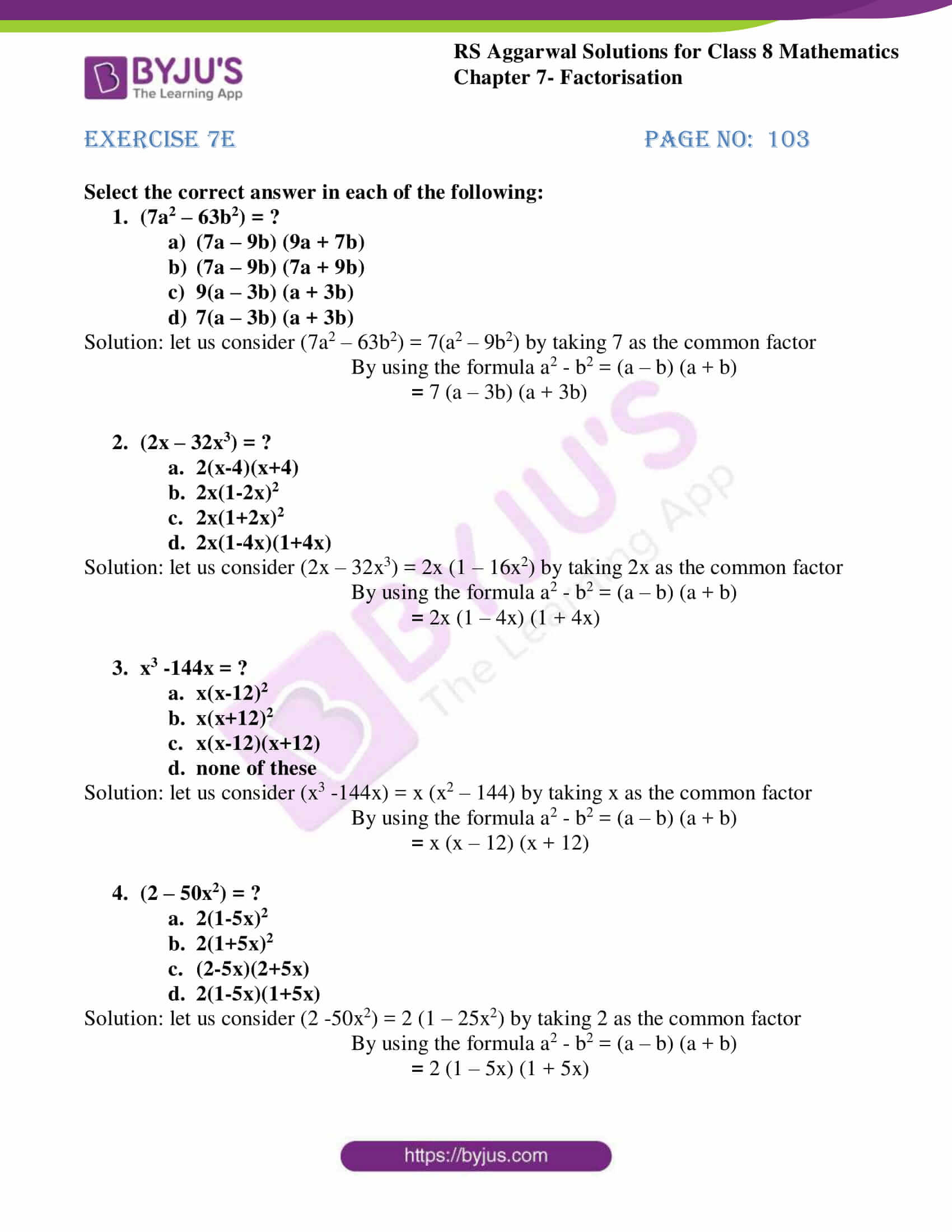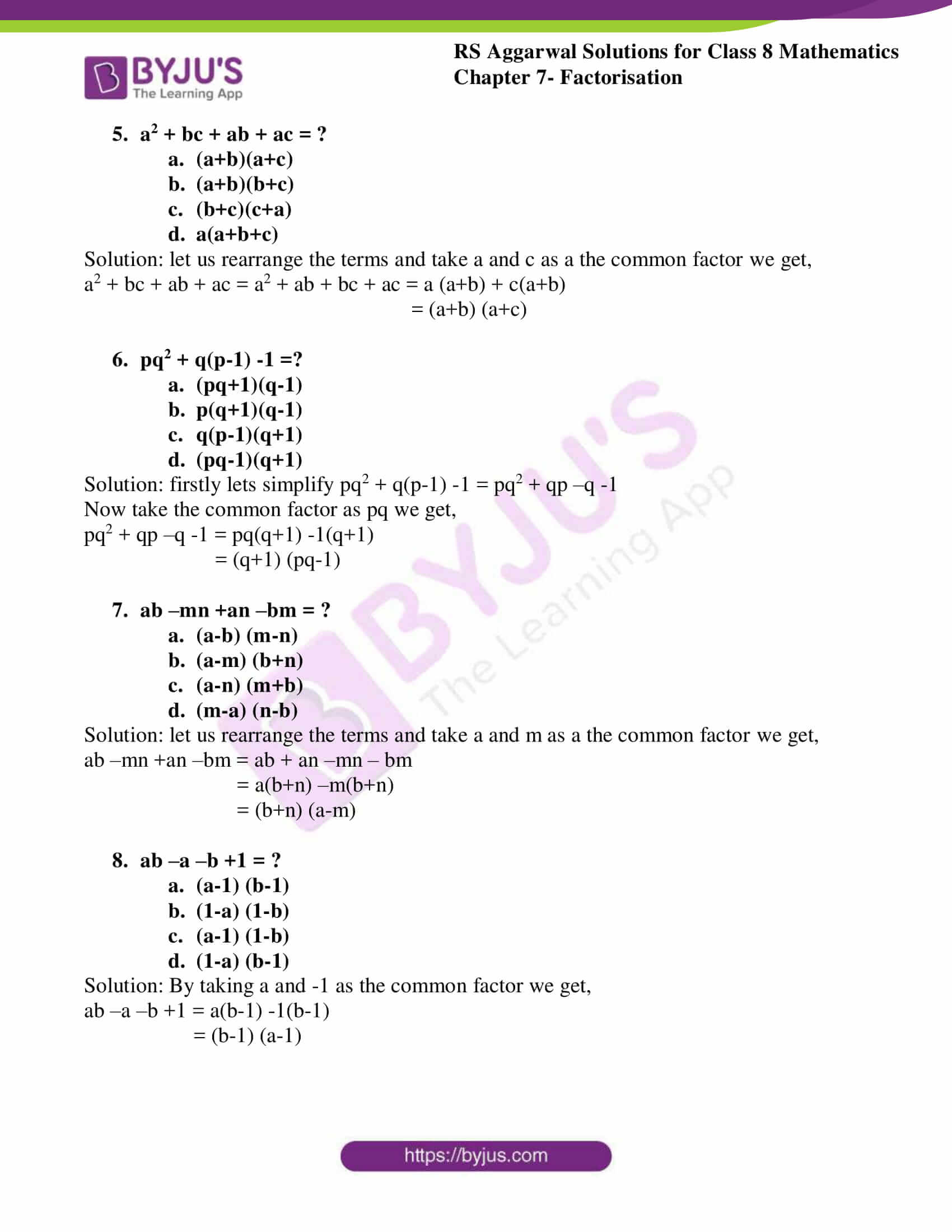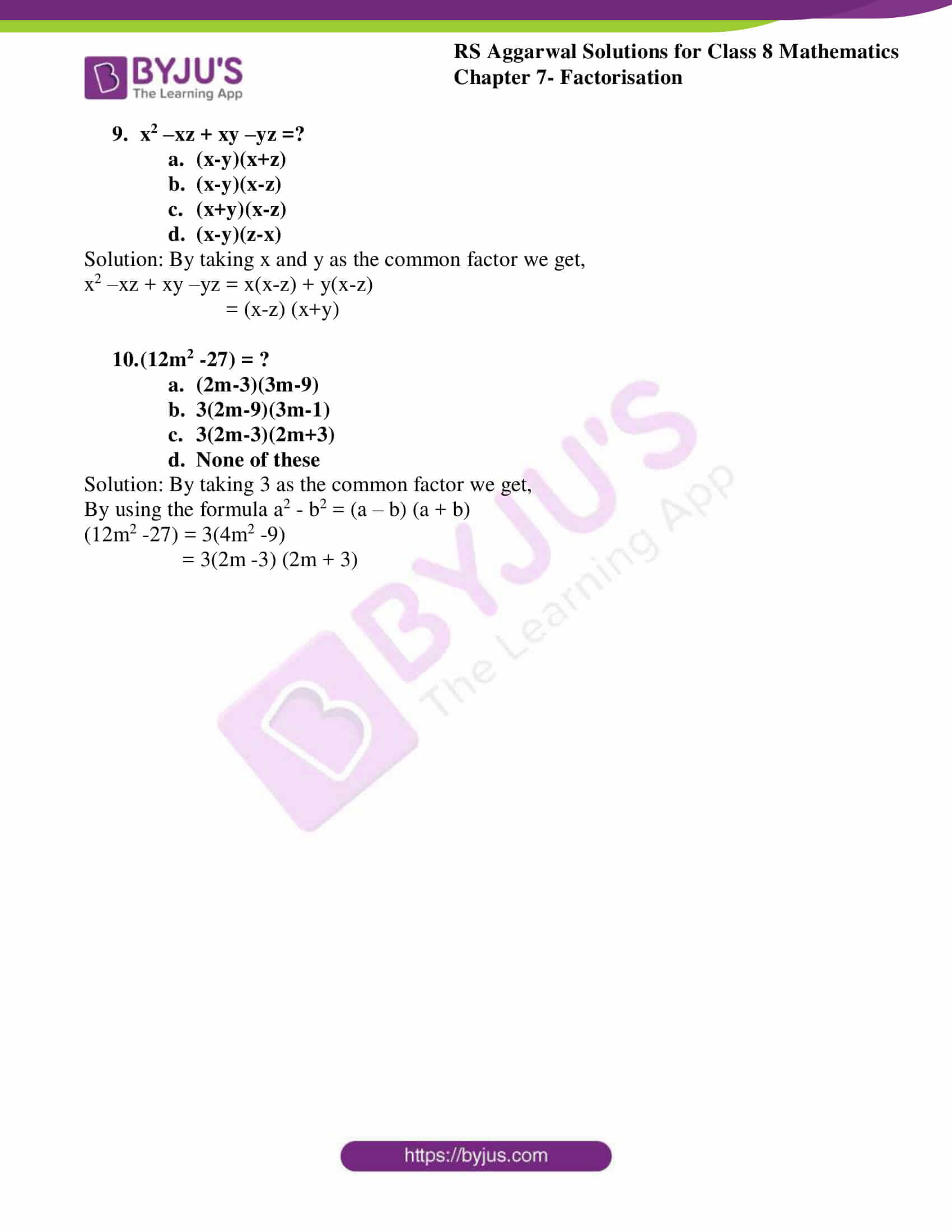# RS Aggarwal Solutions for Class 8 Chapter 7 - Factorisation Exercise 7E

Our expert team has solved the RS Aggarwal Solutions to ensure that the students are precise with their fundamentals by practicing the solutions. Students can refer and download RS Aggarwal Solutions for Class 8 Maths Chapter 7- Exercise 7E, Factorisation from the links provided below. In Exercise 7E of RS Aggarwal Class 8 Maths, we shall brief all the concepts covered in this Chapter i.e. Factors, Factorisation and Factorisation methods.

## Download PDF of RS Aggarwal Solutions for Class 8 Maths Chapter 7- Exercise 7E### Access Answers to RS Aggarwal Solutions for Class 8 Maths Chapter 7- Factorisation Exercise 7E

Select the correct answer in each of the following:

1. (7a2 – 63b2) = ?

1. (7a – 9b) (9a + 7b)
2. (7a – 9b) (7a + 9b)
3. 9(a – 3b) (a + 3b)
4. 7(a – 3b) (a + 3b)

Solution: let us consider (7a2 – 63b2) = 7(a2 – 9b2) by taking 7 as the common factor

By using the formula a2 – b2 = (a – b) (a + b)

= 7 (a – 3b) (a + 3b)

2. (2x – 32x3) = ?

1. 2(x-4)(x+4)
2. 2x(1-2x)2
3. 2x(1+2x)2
4. 2x(1-4x)(1+4x)

Solution: let us consider (2x – 32x3) = 2x (1 – 16x2) by taking 2x as the common factor

By using the formula a2 – b2 = (a – b) (a + b)

= 2x (1 – 4x) (1 + 4x)

3. x3 -144x = ?

1. x(x-12)2
2. x(x+12)2
3. x(x-12)(x+12)
4. none of these

Solution: let us consider (x3 -144x) = x (x2 – 144) by taking x as the common factor

By using the formula a2 – b2 = (a – b) (a + b)

= x (x – 12) (x + 12)

4. (2 – 50x2) = ?

1. 2(1-5x)2
2. 2(1+5x)2
3. (2-5x)(2+5x)
4. 2(1-5x)(1+5x)

Solution: let us consider (2 -50x2) = 2 (1 – 25x2) by taking 2 as the common factor

By using the formula a2 – b2 = (a – b) (a + b)

= 2 (1 – 5x) (1 + 5x)

5. a2 + bc + ab + ac = ?

1. (a+b)(a+c)
2. (a+b)(b+c)
3. (b+c)(c+a)
4. a(a+b+c)

Solution: let us rearrange the terms and take a and c as a the common factor we get,

a2 + bc + ab + ac = a2 + ab + bc + ac = a (a+b) + c(a+b)

= (a+b) (a+c)

6. pq2 + q(p-1) -1 =?

1. (pq+1)(q-1)
2. p(q+1)(q-1)
3. q(p-1)(q+1)
4. (pq-1)(q+1)

Solution: firstly lets simplify pq2 + q(p-1) -1 = pq2 + qp –q -1

Now take the common factor as pq we get,

pq2 + qp –q -1 = pq(q+1) -1(q+1)

= (q+1) (pq-1)

7. ab –mn +an –bm = ?

1. (a-b) (m-n)
2. (a-m) (b+n)
3. (a-n) (m+b)
4. (m-a) (n-b)

Solution: let us rearrange the terms and take a and m as a the common factor we get,

ab –mn +an –bm = ab + an –mn – bm

= a(b+n) –m(b+n)

= (b+n) (a-m)

8. ab –a –b +1 = ?

1. (a-1) (b-1)
2. (1-a) (1-b)
3. (a-1) (1-b)
4. (1-a) (b-1)

Solution: By taking a and -1 as the common factor we get,

ab –a –b +1 = a(b-1) -1(b-1)

= (b-1) (a-1)

9. x2 –xz + xy –yz =?

1. (x-y)(x+z)
2. (x-y)(x-z)
3. (x+y)(x-z)
4. (x-y)(z-x)

Solution: By taking x and y as the common factor we get,

x2 –xz + xy –yz = x(x-z) + y(x-z)

= (x-z) (x+y)

10. (12m2 -27) = ?

1. (2m-3)(3m-9)
2. 3(2m-9)(3m-1)
3. 3(2m-3)(2m+3)
4. None of these

Solution: By taking 3 as the common factor we get,

By using the formula a2 – b2 = (a – b) (a + b)

(12m2 -27) = 3(4m2 -9)

= 3(2m -3) (2m + 3)

## RS Aggarwal Solutions for Class 8 Maths Chapter 7- Exercise 7E

Exercise 7E of RS Aggarwal Class 8, Factorisation. This exercise mainly deals with objective questions related to the entire Chapter. The RS Aggarwal Solutions can help the students practice diligently while learning the fundamentals as it provides all the answers to the questions from the RS Aggarwal textbook. Students are suggested to practice the problems very sincerely, which will help them excel in their exams and maximise their overall percentage. Practising as many times as possible helps in building time management abilities and also boosts the confidence level to achieve high marks.